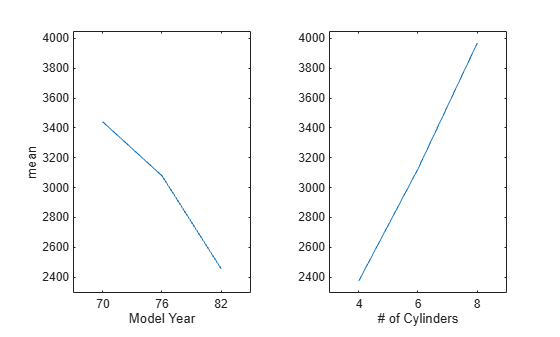Main Content

# maineffectsplot

Main effects plot for grouped data

## Syntax

```maineffectsplot(Y,GROUP) maineffectsplot(Y,GROUP,param1,val1,param2,val2,...) [figh,AXESH] = maineffectsplot(...) ```

## Description

`maineffectsplot(Y,GROUP)` displays main effects plots for the group means of matrix `Y` with groups defined by entries in `GROUP`, which can be a cell array or a matrix. `Y` is a numeric matrix or vector. If `Y` is a matrix, the rows represent different observations and the columns represent replications of each observation. If `GROUP` is a cell array, then each cell of `GROUP` must contain a grouping variable that is a categorical variable, numeric vector, character matrix, string array, or single-column cell array of character vectors. If `GROUP` is a matrix, then its columns represent different grouping variables. Each grouping variable must have the same number of rows as `Y`. The number of grouping variables must be greater than 1.

The display has one subplot per grouping variable, with each subplot showing the group means of `Y` as a function of one grouping variable.

`maineffectsplot(Y,GROUP,param1,val1,param2,val2,...)` specifies one or more of the following name/value pairs:

• `'varnames'` — Grouping variable names in a character matrix, a string array, or a cell array of character vectors, one per grouping variable. Default names are `'X1'`, `'X2'`, ... .

• `'statistic'` — Values that indicate whether the group mean or the group standard deviation should be plotted. Use `'mean'` or `'std'`. The default is `'mean'`. If the value is `'std'`, `Y` is required to have multiple columns.

• `'parent'` — A handle to the figure window for the plots. The default is the current figure window.

`[figh,AXESH] = maineffectsplot(...)` returns the handle `figh` to the figure window and an array of handles `AXESH` to the subplot axes.

## Examples

collapse all

Load the sample data.

`load carsmall;`

Display main effects plots for car weight with two grouping variables, model year and number of cylinders.

```maineffectsplot(Weight,{Model_Year,Cylinders}, ... 'varnames',{'Model Year','# of Cylinders'})```## See Also

### Topics

Introduced in R2006b

Download ebook